# 1687 Fly Me to the Moon!

### Today’s Puzzle:

A witch flying on a broomstick in front of a bright full moon is a common Halloween image. Here is a level 4 puzzle shaped like a broom. If you succeed in solving it, you might just feel like you are flying to the moon, too. Just write the numbers from 1 to 12 in both the first column and in the top row so that those numbers and the given clues form a multiplication table. Best Witches!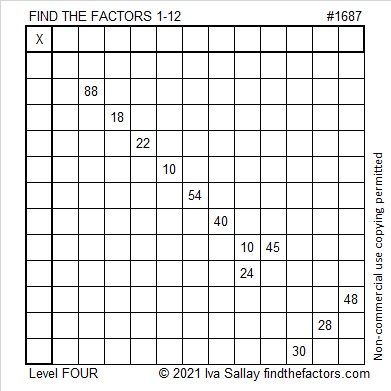### Factors of 1687:

1687 is divisible by 7 because 16 is the double of 8, and the last digit is 7.
217, 427, 637, 847, 1057, 1267, 1477, 1687, 1897 are all divisible by 7.

• 1687 is a composite number.
• Prime factorization: 1687 = 7 × 241.
• 1687 has no exponents greater than 1 in its prime factorization, so √1687 cannot be simplified.
• The exponents in the prime factorization are 1 and 1. Adding one to each exponent and multiplying we get (1 + 1)(1 + 1) = 2 × 2 = 4. Therefore 1687 has exactly 4 factors.
• The factors of 1687 are outlined with their factor pair partners in the graphic below.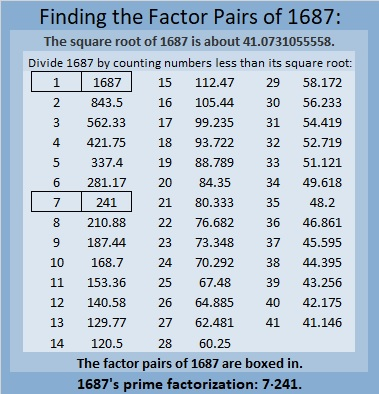### More About the Number 1687:

1687 is the hypotenuse of a Pythagorean triple:
840-1463-1687, which is 7 times (120-209-241).

# 1686 Some Candy Corn for You to Chew on

### Today’s Puzzle:

Candy corn probably isn’t your favorite Halloween treat, but this candy corn puzzle could give you something satisfying to chew on. Give it a try!

Find the common factor of 33 and 66, write the factors in the appropriate cells. Since this is a level 3 puzzle, you can then work from the top of the puzzle row by row until you have found all the factors. The numbers from 1 to 12 must appear once in both the first column and the top row.Here’s the same puzzle without any color if you prefer: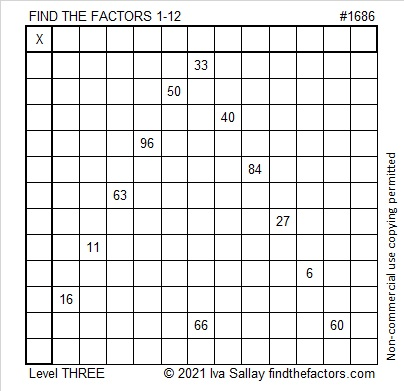### Factors of 1686:

• 1686 is a composite number.
• Prime factorization: 1686 = 2 × 3 × 281.
• 1686 has no exponents greater than 1 in its prime factorization, so √1686 cannot be simplified.
• The exponents in the prime factorization are 1, 1, and 1. Adding one to each exponent and multiplying we get (1 + 1)(1 + 1)(1 + 1) = 2 × 2 × 2 = 8. Therefore 1686 has exactly 8 factors.
• The factors of 1686 are outlined with their factor pair partners in the graphic below.### More about the number 1686:

1686 is the hypotenuse of a Pythagorean triple:
960-1386-1686, which is 6 times (160-231-281).

1686 is also a leg in these two Pythagorean triples:
1686-710648-710650, calculated from 2(843)(1), 843² – 1², 843² + 1² and
1686-78952-78970, calculated from 2(281)(3), 281² – 3², 281² – 3².

# 1532 Don’t Let This Puzzle Spook You!

### Today’s Puzzle:

If this little ghost prowls your neighborhood this Halloween, don’t let it spook you. Sure, it is a level 6 puzzle, but if you stick to using logic from start to finish, you’ll know the most about this ghost!Here’s the same puzzle minus the embellishments: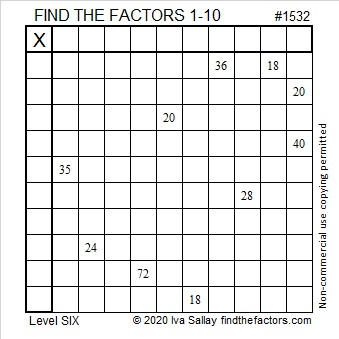If you know what a normal distribution is, then you should enjoy this statistics joke:

### Factors of 1532:

• 1532 is a composite number.
• Prime factorization: 1532 = 2 × 2 × 383, which can be written 1532 = 2² × 383.
• 1532 has at least one exponent greater than 1 in its prime factorization so √1532 can be simplified. Taking the factor pair from the factor pair table below with the largest square number factor, we get √1532 = (√4)(√383) = 2√383.
• The exponents in the prime factorization are 2 and 1. Adding one to each exponent and multiplying we get (2 + 1)(1 + 1) = 3 × 2 = 6. Therefore 1532 has exactly 6 factors.
• The factors of 1532 are outlined with their factor pair partners in the graphic below.### More about the Number 1532:

1532 = 2 × 383 × 2. That factorization looks the same frontwards or backward.

1532 can be written as the difference of two squares:
384² – 382² = 1532.

# 1529 Pointy Hat

#### Today’s Puzzle:

A pointy hat is part of many different Halloween costumes: Wizards, Witches, Medieval Princesses, and Clowns come to my mind. Today’s puzzle looks like a pointy hat. Use logic to work your magic in solving it! As always, there is only one solution.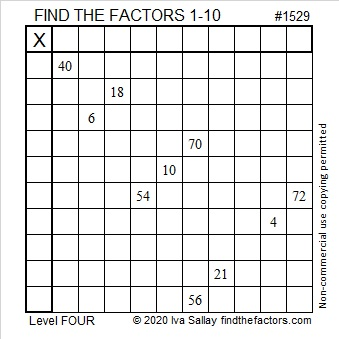### Factors of 1529:

Look at this math fact using the digits of 1529:
1 – 5 + 2 – 9 = -11, a number divisible by 11, so 1529 is divisible by 11.

• 1529 is a composite number.
• Prime factorization: 1529 = 11 × 139.
• 1529 has no exponents greater than 1 in its prime factorization, so √1529 cannot be simplified.
• The exponents in the prime factorization are 1 and 1. Adding one to each exponent and multiplying we get (1 + 1)(1 + 1) = 2 × 2 = 4. Therefore 1529 has exactly 4 factors.
• The factors of 1529 are outlined with their factor pair partners in the graphic below.### More about the Number 1529:

1529 is the difference of two squares in two different ways:
765² – 764²  = 1529, and
75² – 64²  = 1529.

# 1528 Candy Corn

### Today’s Puzzle:

To solve this Level 3 Candy Corn Halloween puzzle, first, find the factors that will work with the clues in the top and bottom rows. Then work you way down row by row filling in factors as you go.

When you get to the 8 in this puzzle, will the factors be 8 × 1 or 4 × 2? Two of those factors will be eliminated because they already appear in the first column. The other two remain possibilities, but one of those factors cannot appear in any other place in that first column, so that is the one you will want to choose. Have a sweet time solving this puzzle!Here is a plain version of the same puzzle: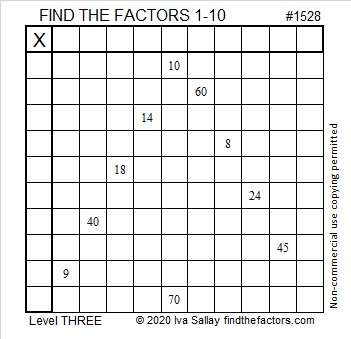### Factors of 1528:

1528 is divisible by two because it is even.

1528 is divisible by four because its last two digits (in the same order) make a number, 28, which is divisible by 4.

Can 1528 be evenly divided by 8? Yes. Here’s a quick way to know: 28 is divisible by 4, but not by 8, AND 5 is odd, so 1528 is divisible by 8, as is every other number ending in 528.

• 1528 is a composite number.
• Prime factorization: 1528 = 2 × 2 × 2 × 191, which can be written 1528 = 2³ × 191.
• 1528 has at least one exponent greater than 1 in its prime factorization so √1528 can be simplified. Taking the factor pair from the factor pair table below with the largest square number factor, we get √1528 = (√4)(√382) = 2√382.
• The exponents in the prime factorization are 3 and 1. Adding one to each exponent and multiplying we get (3 + 1)(1 + 1) = 4 × 2 = 8. Therefore 1528 has exactly 8 factors.
• The factors of 1528 are outlined with their factor pair partners in the graphic below.### Another Fact about the Number 1528:

Since 1528 is divisible by 8 but not by 16, it can be written as the sum of 16 consecutive numbers:
88+89+90+91+92+93+94+95+96+97+98+99+100+101+102+103=1528.

Note that 95 + 96 = 191, and 8 × 191 = 1528.
Likewise, 94 + 97 = 1528,
93 + 98 = 1528, and so forth until we get to…
88 + 103 = 1528.

# 1527 Not a Haunted House

### Today’s Puzzle:

We see plenty of pumpkins on doorsteps this time of year, so I put a few in this puzzle. The puzzle looks a bit like a house, but certainly not a haunted house. Can you write the numbers from 1 to 10 in both the first column and the top row so that the given number clues are the products of those numbers?

Here’s the same puzzle without the added colorful embellishments:### Factors of 1527:

• 1527 is a composite number.
• Prime factorization: 1527 = 3 × 509.
• 1527 has no exponents greater than 1 in its prime factorization, so √1527 cannot be simplified.
• The exponents in the prime factorization are 1, and 1. Adding one to each exponent and multiplying we get (1 + 1)(1 + 1) = 2 × 2 = 4. Therefore 1527 has exactly 4 factors.
• The factors of 1527 are outlined with their factor pair partners in the graphic below.### Another Fact about the Number 1527:

1527 is the hypotenuse of a Pythagorean triple:
660-1377-1527, which is 3 times (220-459-509).

# 1526 Grave Marker

### Today’s Puzzle:

It’s almost Halloween! This is my favorite kind of grave marker, one that is really just a Find the Factors puzzle in disguise. It’s only a level one, so it isn’t very tricky. I hope you find it a real treat!Here’s the same puzzle but requiring less ink to print:Neighbors have decorated part of their yard to look like a mini graveyard for Halloween. I think my grave marker would fit right in!### Factors of 1526:

• 1526 is a composite number.
• Prime factorization: 1526 = 2 × 7 × 109.
• 1526 has no exponents greater than 1 in its prime factorization, so √1526 cannot be simplified.
• The exponents in the prime factorization are 1, 1, and 1. Adding one to each exponent and multiplying we get (1 + 1)(1 + 1)(1 + 1) = 2 × 2 × 2 = 8. Therefore 1526 has exactly 8 factors.
• The factors of 1526 are outlined with their factor pair partners in the graphic below.### Another Fact about the Number 1526:

1526 is the hypotenuse of a Pythagorean triple:
840-1274-1526 which is 14 times (60-91-109)

# 1427 Mysterious Cat

This mysterious Halloween cat shares twelve clues that can help you solve its puzzle. Each clue is the products of two factors from 1 to 12 multiplied together. Will you be able to solve its mystery?Print the puzzles or type the solution in this excel file: 12 Factors 1419-1429

Now I’ll tell you a little bit about the puzzle number, 1427:

• 1427 is a prime number.
• Prime factorization: 1427 is prime.
• 1427 has no exponents greater than 1 in its prime factorization, so √1427 cannot be simplified.
• The exponent in the prime factorization is 1. Adding one to that exponent we get (1 + 1) = 2. Therefore 1427 has exactly 2 factors.
• The factors of 1427 are outlined with their factor pair partners in the graphic below.

How do we know that 1427 is a prime number? If 1427 were not a prime number, then it would be divisible by at least one prime number less than or equal to √1427. Since 1427 cannot be divided evenly by 2, 3, 5, 7, 11, 13, 17, 19, 23, 29, 31, or 37, we know that 1427 is a prime number.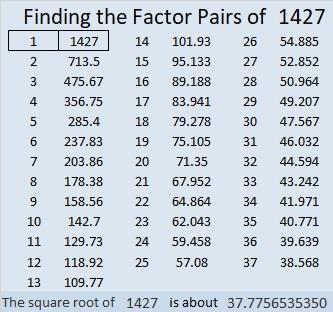1427 is 272 in BASE 25 because 2(25²) + 7(25) + 2(1) = 1427

# 1425 Jack O’Lantern

Most of my puzzles don’t have as many clues as this Jack O’lantern puzzle has. Those extra clues could make it easier to solve. On the other hand, some of the clues might still be tricky.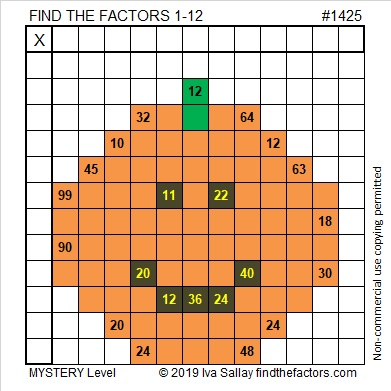Print the puzzles or type the solution in this excel file: 12 Factors 1419-1429

Here are some facts about the puzzle number, 1425:

• 1425 is a composite number.
• Prime factorization: 1425 = 3 × 5 × 5 × 19, which can be written 1425 = 3 × 5² × 19.
• 1425 has at least one exponent greater than 1 in its prime factorization so √1425 can be simplified. Taking the factor pair from the factor pair table below with the largest square number factor, we get √1425 = (√25)(√57) = 5√57.
• The exponents in the prime factorization are 1, 2, and 1. Adding one to each exponent and multiplying we get (1 + 1)(2 + 1)(1 + 1) = 2 × 3 × 2 = 12. Therefore 1425 has exactly 12 factors.
• The factors of 1425 are outlined with their factor pair partners in the graphic below.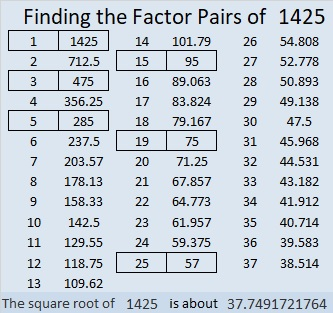1425 is the hypotenuse of TWO Pythagorean triples:
399-1368-1425 which is (7-24-25) times 57
855-1140-1425 which is (3-4-5) times 285

# 1269 Tangram Witch Puzzle

If you have the seven tangram pieces, then you can create this Halloween witch riding across the moon (or a paper plate). Best Witches creating all kinds of things with those fabulous tiles!In case you would like to know some facts about the number 1269, here’s what I’ve learned:

• 1269 is a composite number.
• Prime factorization: 1269 = 3 × 3 × 3 × 47, which can be written 1269 = 3³ × 47
• The exponents in the prime factorization are 3 and 1. Adding one to each and multiplying we get (3 + 1)(1 + 1) = 4 × 2 = 8. Therefore 1269 has exactly 8 factors.
• Factors of 1269: 1, 3, 9, 27, 47, 141, 423, 1269
• Factor pairs: 1269 = 1 × 1269, 3 × 423, 9 × 141, or 27 × 47
• Taking the factor pair with the largest square number factor, we get √1269 = (√9)(√141) = 3√141 ≈ 35.62303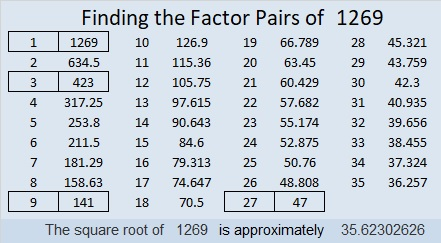1269 is the difference of two squares four different ways:
37² – 10² = 1269
75² – 66² = 1269
213² – 210² = 1269
635² – 634² = 1269